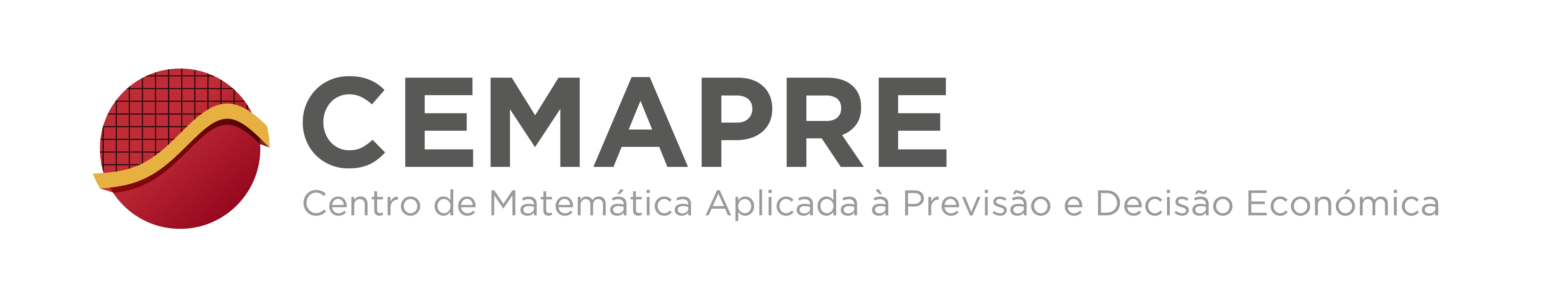## CEMAPRE Seminar

### Dynamics of 2-interval piecewise affine maps and Hecke-Mahler series

Arnaldo Nogueira
(Aix-Marseille Université)

Abstract: Let $f=\displaystyle f_{\lambda,\delta,\mu}$ be a family of interval maps described by the below graph in terms of the parameters $\lambda,\delta,\mu$. Any map $f$ has a rotation number and its dynamics will be described with the help of two functions of the three parameters whose definitions use Hecke-Mahler series. As a consequence of our approach, we prove that whenever the parameters $\lambda,\delta$ e $\mu$ are algebraic numbers the rotation number takes a rational value. This result extends our previous theorem about the case where $f$ is a circle contracted rotation which means that the parameter $\mu=1$. {\it (The talk is based on a joint work with Michel Laurent.)

Wednesday, December 18, 2019
Time: 14h00
Room: Sala Novo Banco, Edificio Quelhas, ISEG
http://cemapre.iseg.ulisboa.pt/seminars/cemapre/